﻿ 基于蓝牙传输的智能胎动检测系统设计

# 基于蓝牙传输的智能胎动检测系统设计Design of Intelligent Fetal Movement Detection System Based on Bluetooth Transmission

Abstract: In this paper, based on the fetal movement monitoring to confirm the rigidity of the fetus in a normal state, a set of intelligent fetal movement detection system based on Bluetooth transmission is designed and implemented. The hardware terminal + mobile APP monitoring mode is adopted, the hardware terminal realizes fetal movement signal acquisition and processing; the mobile phone APP realizes the feature point recognition of the fetal movement signal, and then performs fetal movement counting. The system can effectively realize the automatic monitoring of fetal movement, and can detect fetal movement abnormality in time and reduce the incidence and mortality of perinatal infants, which is of great significance for improving the level of prenatal and postnatal care.

1. 引言

2. 系统测量原理Figure 1. Euler

${x}^{\beta }={C}_{\alpha }^{\beta }{x}^{\alpha }$ (1)

${C}_{\beta }^{\alpha }=\left[\begin{array}{ccc}\mathrm{cos}\theta \mathrm{cos}\psi & -\mathrm{cos}\varphi \mathrm{sin}\psi +\mathrm{sin}\varphi \mathrm{sin}\theta \mathrm{cos}\psi & \mathrm{sin}\varphi \mathrm{sin}\psi +\mathrm{cos}\varphi \mathrm{sin}\theta \mathrm{cos}\psi \\ \mathrm{cos}\theta \mathrm{sin}\psi & \mathrm{cos}\varphi \mathrm{cos}\psi +\mathrm{sin}\varphi \mathrm{sin}\theta \mathrm{sin}\psi & -\mathrm{sin}\varphi \mathrm{cos}\psi +\mathrm{cos}\varphi \mathrm{sin}\theta \mathrm{sin}\psi \\ -\mathrm{sin}\theta & \mathrm{sin}\varphi \mathrm{cos}\theta & \mathrm{cos}\varphi \mathrm{cos}\theta \end{array}\right]$ (2)

$|a|=\sqrt{{a}_{x}^{2}+{a}_{y}^{2}+{a}_{z}^{2}}$ (3)

3. 系统总体方案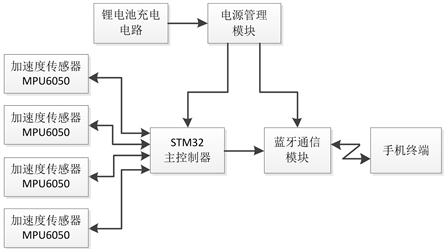Figure 2. System block diagram

4. 系统硬件设计

4.1. 胎动传感器的选择

1) 超声多普勒

2) 压力传感器

3) 加速度传感器

4.2. 胎动采集模块设计

4.3. 胎动采集模块的布置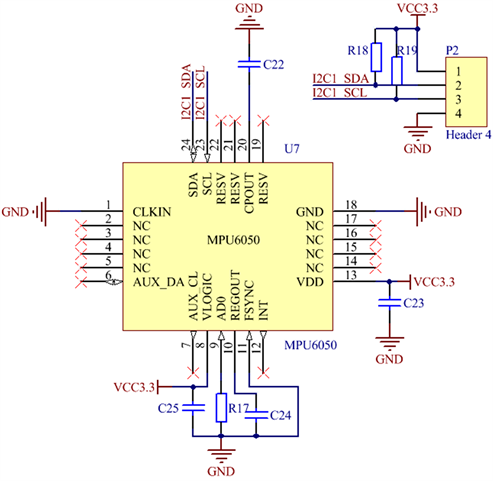Figure 3. MPU6050 connection circuit diagram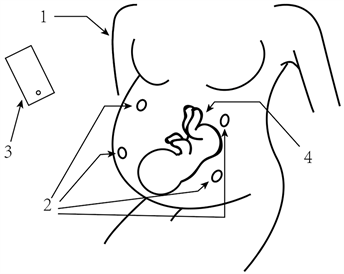Figure 4. Acceleration sensors’ array diagram

4.4. 数据传输模块设计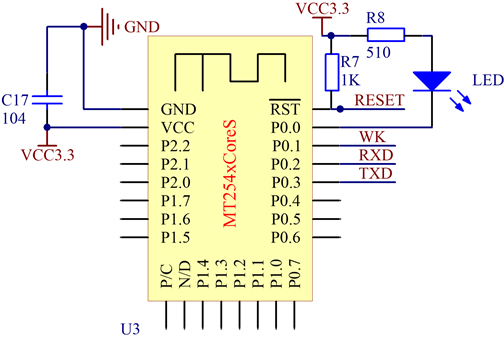Figure 5. Bluetooth module circuit schematic

4.5. 单片机核心处理模块设计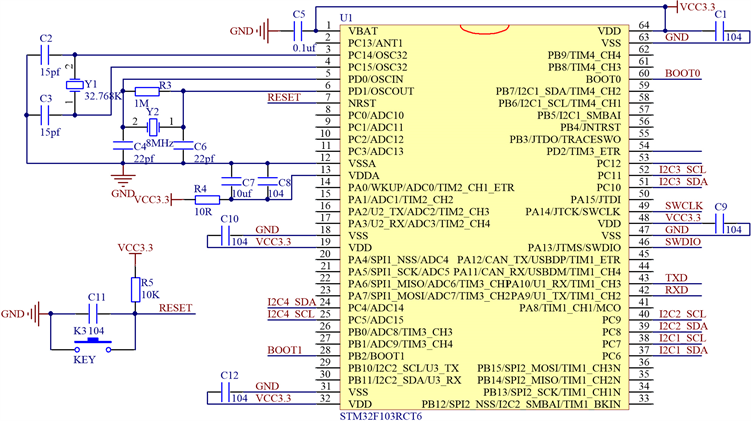Figure 6. STM32 main control circuit schematic

4.6. 供电电源设计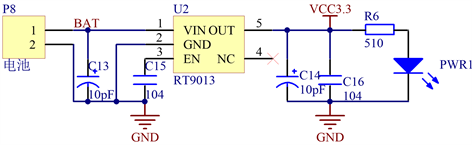Figure 7. 3.3 V regulator circuit schematic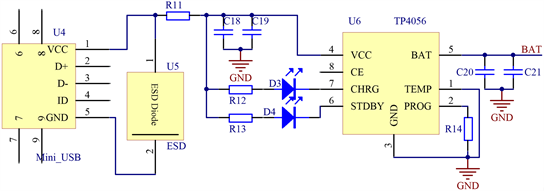Figure 8. Lithium battery charging circuit schematic

4.7. 系统硬件设计实物图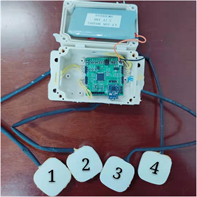Figure 9. System hardware design physical map

5. 系统软件设计

5.1. 终端软件设计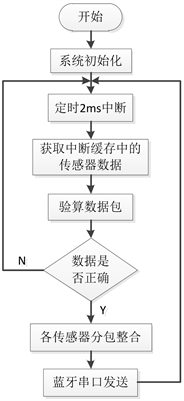Figure 10. Terminal signal acquisition and processing

5.2. 手机APP设计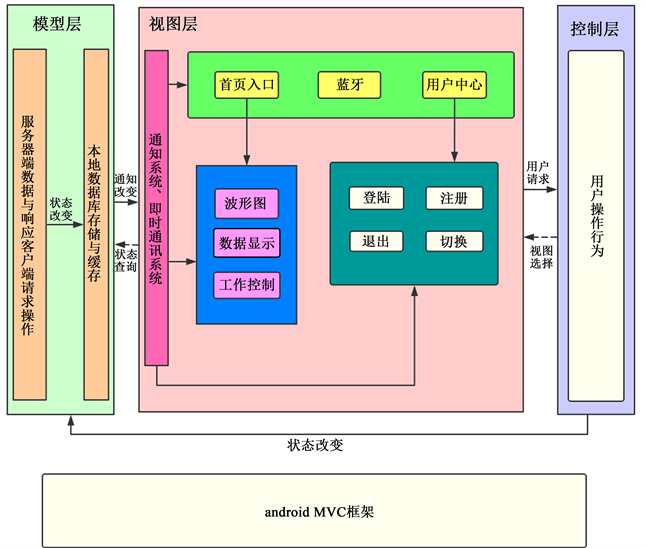Figure 11. Android MVC framework

1) 通过Android的蓝牙接口从硬件终端获得一个数据包，进行解包与数据处理，选定一段信号；

2) 设定一个基准阈值，判断信号最大值是否大于阈值，若没有再取下一个数据包再处理判断；

3) 对阈值判断后的信号进行一阶微分： $dx\left(n-1\right)=x\left(n\right)-x\left(n-1\right)$，其中n为整数；

4) 根据 $dx\left(n\right)*dx\left(n-1\right)$ 的值来判断波峰与波谷，如果其值小于0，则记录该点(dx(n)为0时，记为−1)；

5) 对所有的波峰与波谷进行识别，从峰点开始检测，如果第一个点是谷点，则跳过。寻找所有满足比左邻近最小谷点值(初始值为信号最小值，指从上次检测到的有效峰值点开始，到当前峰点之间的最小谷值点)、比右邻近谷点都高于一定阈值的有效峰点，并且在一个有效峰值检测过程中，检测到的峰值为两次有效检测所有可能的峰点中的最大值点。有效则保留，无效则丢弃，进行下一轮峰值检测。

6. 系统测试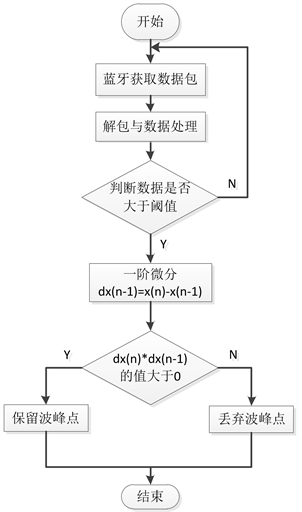Figure 12. Peak algorithm detection step diagram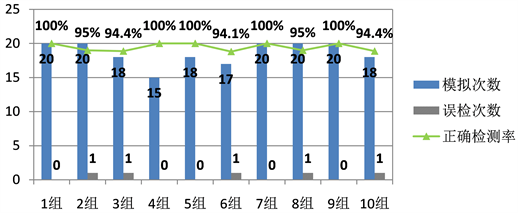Figure 13. Simulation test result

7. 总结

NOTES

*通讯作者。

 吴益珍. 胎动计数的临床意义[J]. 实用妇科与产科杂志, 1987(6): 285-286.

 习海燕. 面向社区家庭的母婴健康监护系统软件设计[D]: [硕士学位论文]. 广州: 南方医科大学, 2015.

 赛西亚, 王艳萍. 超声胎儿生物物理评分及多普勒监测胎儿血流在晚期妊娠的应用[J]. 中国超声诊断杂志, 2003(4): 318-319.

 Karduna, A.R., Mcclure, P.W. and Michener, L.A. (2000) Scapular Kinematics: Effects of Altering the Euler Angle Sequence of Rota-tions. Journal of Biomechanics, 33, 1063-1068.
https://doi.org/10.1016/S0021-9290(00)00078-6

 Bar-Itzhack, I.Y. and Fegley, K.A. (1969) Orthogonalization Techniques of a Direction Cosine Matrix. IEEE Transactions on Aerospace Electronic Systems, AES-5, 798-804.
https://doi.org/10.1109/TAES.1969.309878

 Liu, R., Zhou, J.Z., Liu, M. and Hou, X.F. (2007) A Wearable Acceleration Sensor System for Gait Recognition. 2nd IEEE Conference on Industrial Electronics and Applications, ICIEA.
https://doi.org/10.1109/ICIEA.2007.4318894

Top